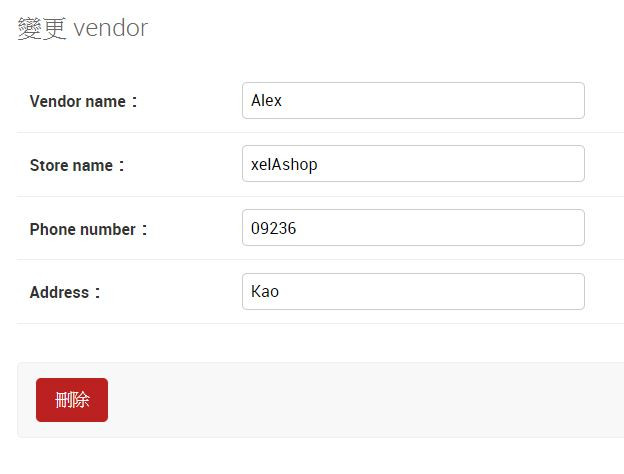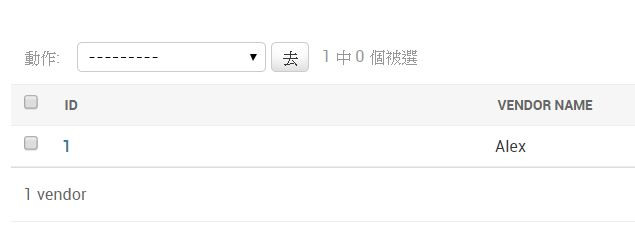#2019 iT 邦幫忙鐵人賽DAY 10
0
Modern Web

## Day10 : Models & Admin (1)

Day9 介紹了透過Shell來完成CRUD的功能，而這種將關聯式資料庫映射到物件導向(OO)

``````>>> from vendor.models import Vendor
>>> v1 = Vendor.objects.get(id=1)
>>> v1
<Vendor: Vendor object (1)>
>>>
``````

``````from django.db import models

class Vendor(models.Model):
vendor_name = models.CharField(max_length = 20) # 攤販的名稱
store_name = models.CharField(max_length = 10) # 攤販店家的名稱
phone_number = models.CharField(max_length = 20) # 攤販的電話號碼
address = models.CharField(max_length = 100) # 攤販的地址

# 覆寫 __str__
def __str__(self):
return self.vendor_name

class Food(models.Model):
food_name = models.CharField(max_length = 30) # 食物名稱
price_name = models.DecimalField(max_digits = 3, decimal_places=0) # 食物價錢
food_vendor = models.ForeignKey(Vendor, on_delete=models.CASCADE) # 代表這食物是由哪一個攤販所做的

def __str__(self):
return self.food_name
``````

``````>>> from vendor.models import Vendor
>>> v1 = Vendor.objects.get(id=1)
>>> v1
<Vendor: Alex>
>>>
````````````from django.db import models
# 新增

class Vendor(models.Model):
vendor_name = models.CharField(max_length = 20) # 攤販的名稱
store_name = models.CharField(max_length = 10) # 攤販店家的名稱
phone_number = models.CharField(max_length = 20) # 攤販的電話號碼
address = models.CharField(max_length = 100) # 攤販的地址

def __str__(self):
return self.vendor_name

...中間略

# 新增
list_display = ('id', 'vendor_name')
``````

1. 新增 `from django.contrib import admin`

``````from django.contrib import admin
from .models import Vendor, Food, VendorAdmbin

``````0